# NCERT Solutions for Class 10 Science Electricity Part 1## myCBSEguide App

Complete Guide for CBSE Students

NCERT Solutions, NCERT Exemplars, Revison Notes, Free Videos, CBSE Papers, MCQ Tests & more.

NCERT solutions for Class 10 Science Electricity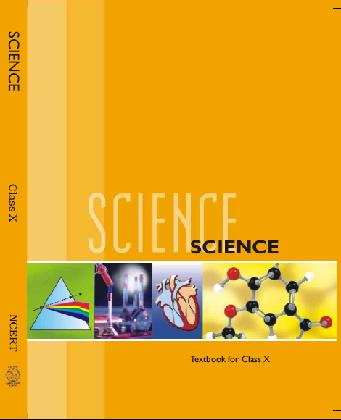## NCERT Class 10 Science Chapter wise Solutions

• 1 – Chemical Reactions and Equations
• 2 – Acids, Bases and Salts
• 3 – Metals and Non-metals
• 4 – Carbon and Its Compounds
• 5 – Periodic Classification of Elements
• 6 – Life Processes
• 7 – Control and Coordination
• 8 – How do Organisms Reproduce?
• 9 – Heredity and Evolution
• 10 – Light Reflection and Refraction
• 11 – Human Eye and Colourful World
• 12 – Electricity
• 13 – Magnetic Effects of Electric Current
• 14 – Sources of Energy
• 15 – Our Environment
• 16 – Management of Natural Resources

## NCERT Solutions for Class 10 Science Electricity Part 1

###### 1. What does an electric circuit mean?

Ans. An electric circuit is a continuous and closed path of an electric current. If the electric circuit complete, current can flow through the circuit.

###### 2. Define the unit of current.

Ans. SI unit of electric current is Ampere. Current is said to be 1 ampere, if 1 coulomb charge flows per second across a cross-section of conductor.

###### 3. Calculate the number of electrons constituting one coulomb of charge.

Ans. Charge on one electron = 1.6 x 10-19 coulomb.

No of electron in one coulomb of charge = 1/1.6 x 10-19

= 6.25 x 1018

Page No. 202

Ans. Battery

###### 2. What is meant by saying that the potential difference between two points is 1 v?

Ans. The potential difference between two points is said to be 1 volt, if 1 joule of work is to be done for moving charge of 1 coulomb from one point to another.

###### 3. How much energy is given to each coulomb of charge passing through a 6-volt battery?

Ans. Potential difference (V) = 6 V

Charge (Q) = 1 C

Energy = total work done (W) = Q x V = 1×6 = 6 joule.

NCERT Solutions for Class 10 Science Electricity Part 1
1. On what factor does the resistance of a conductor depend?

Ans. The resistance of a conductor depends on

i. length of conductor (l)

ii. Area of cross-section (A)

iii. Temperature

iv. Nature of material used to make conductor

###### 2. Will current flow more easily through a thick wire or a thin wire of the same material, when connected to the same source? Why?

Ans. The current flows more easily through a thick wire as compared to thin wire of the same material, when connected to the same source. It is due to the reason that resistance increases with decrease in thickness.

NCERT Solutions for Class 10 Science Electricity Part 1

###### 3. Let the resistance of an electric component remains constant while the potential difference across the two ends of the component decreases to half of its former value. What change will occur in the current through it?

Ans. It is given that resistance R of the electrical component remains constant but the potential difference across the ends of the component decreases to half of its value.

Hence, as per Ohm’s law, new current also decreases to half of its original value.

###### 4. Why are coils of electric toasters and electric irons made of an alloy rather than a pure metal?

Ans. Coils of electric toasters and electric irons are made of an alloy due to the following reasons:

i. Resistivity of an alloy is generally higher than that of pure metal.

ii. At high temperature, an alloy does not oxidize readily. Hence, coil of an alloy has longer life.

###### 5. Use the data in Table 12.2 to answer the following:

(a) Which among iron and mercury is a better conductor?

(b) Which material is the best conductor?

Ans. (a) Iron is the better conductor than mercury.

(b) Silver is the best conductor because its resistivity is the least.

NCERT Solutions for Class 10 Science Electricity Part 1

###### 1. Draw a schematic diagram of a circuit consisting of a battery of three cells of 2 V, each, a 5 Ώ resistor, 8 Ώ resistors and a 12 Ώ and a plug key, all connected in series.

Ans. The schematic diagram of circuit is as follows: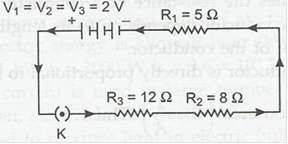NCERT Solutions for Class 10 Science Electricity Part 1

###### 2. Redraw the circuit of question 1, putting in an ammeter to measure the current through the resistors and a voltmeter to measure the potential difference across the 12 Ώ resistors. What would be the reading in the ammeter and voltmeter?

Ans.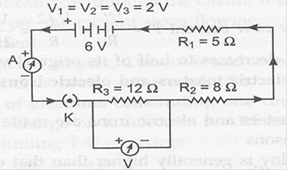Here ammeter A has been joined in series of circuit and voltmeter V is joined in parallel to 12 ohms’ resistor.

Total voltage of battery V = 3×2 = 6 V.

Total resistance R = R1+ R2+ R3 = 5 Ω +8 Ω +12 Ω = 25 Ω

Ammeter reading (current) = I = V/R = 6/25 = 0.24 A.

Voltmeter reading = IR = 0.24 x 12 = 2.88 V.

NCERT Solutions for Class 10 Science Electricity Part 1

###### 1. Judge the equivalent resistance when the following are connected in parallel:

(a) 1 Ω and 106 Ω

(b) 1 Ω, 103 Ω and 106 Ω

Ans. When the resistances are joined in parallel, the resultant resistance in parallel arrangement is given by:

1/R = 1/R1 + 1/R2 + 1/R3 —–

(a) 1/R = 1/1+ 1/106 = 1+ 10-6

R = 1 Ω

(b) 1/R = 1/1 + 1/103 + 1/106 = 1 + 10-3 + 10-6

R= 1 Ω

NCERT Solutions for Class 10 Science Electricity Part 1

###### 2. An electric lamp of 100 Ω, a toaster of resistance 50 Ω and a water filter of resistance 500 Ω are connected in parallel to a 220 V source. What is the resistance of an electric iron connected to the same source that takes as much current as all three appliances, and what is the current through it?

Ans. Here, voltage (V) = 220 V

R1 = 100 Ω, R2 = 50 Ω and R3 = 500 Ω

1/R = 1/R1 + 1/R2 + 1/R3 —–

1/R = 1/100+1/50 +1/500 = 16/500

R = 500/16 = 31.25 Ω

The resistance of electric iron, which draws as much current as all three appliances take together = R = 31.25 Ω.

Current passing through electric iron (I) = V/R = 220/31.25 = 7.04 A.

NCERT Solutions for Class 10 Science Electricity Part 1

###### 3. What are the advantages of connecting electrical devices in parallel with the battery instead of connecting them in series?

Ans. Advantage of connecting electrical devices in parallel with the battery are as follows:

(i) Voltage across each connecting electrical device is same and device take current as per its resistance.

(ii) Separate on/off switches can be applied across each device.

(iii) Total resistance in parallel circuit decreases, hence, a great current may be drawn from cell.

(iv) If one electrical device is damaged; then other devices continue to work properly.

###### 4. How can three resistors of resistance 2 Ω, 3 Ω and 6 Ω be connected to give a total resistance of (a) 4 Ω (b) 9 Ω?

Ans. (a) If we connect resistance of 3 Ω and 6 Ω in parallel and then resistance of 2 Ω is connected in series of the combination, then total resistance of combination is 4 Ω.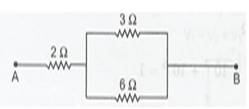###### (b) If all the three resistance are joined in parallel the resultant resistance will be 3 Ω.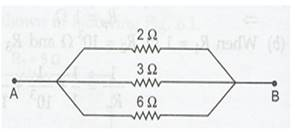NCERT Solutions for Class 10 Science Electricity Part 1

###### 5. What is (a) the highest, (b) the lowest total resistance that can be secured by combination of four resistances of 4 Ω, 8 Ω, 12 Ω and 24 Ω?

Ans. (a) To obtain highest resistance, all the four resistances must be connected in series arrangement. In that case resultant R = R1 + R2 + R3

= 4+8+12 48 Ω

(b) To obtain lowest resistance, all the four resistance must be connected in parallel arrangement. 1/R = 1/R1 + 1/R2 + 1/R3

= 1/4 +1/8 +1/12 + 1/24 = 12/24 Ω

= 24/12 = 2 Ω

NCERT Solutions for Class 10 Science Electricity Part 1

###### 1. Why does the cord of an electric heater not glow while the heating element does?

Ans. Cord of heater and electric heater are joined in series and carry same current when joined to voltage source. As resistance of cord is extremely small as compared to that of heater element. hence, heat produced is extremely small in cord but much larger in heater element. So, the heating element begins to glow but cord does not glow.

###### 2. Compute the heat generated while transferring 96000 coulomb of charge in one hour through a potential difference of 50 V.

Ans. Charge transferred (Q) = 96000 C, time = 1 hour = 60 x 60 = 3600 s and potential difference (V) = 50 V.

Heat generated (H) = VIt = V.Q = 50 x 96000 = 4800000 j = 4.8 x 106 j.

###### 3. An electric iron of resistance 20 takes a current of 5 A. Calculate the heat developed in 30 s.

Ans. Resistance of electric iron (R) = 20 Ω, current (I) = 5 A and time = 30 s.

Heat generated (H) = I2Rt = 52 x 20 x 30 = 15000 j.

NCERT Solutions for Class 10 Science Electricity Part 1

###### 1. What determines the rate at which energy is delivered by a current?

Ans. Electric power determines the rate at which energy is delivered by a current.

NCERT Solutions for Class 10 Science Electricity Part 1

###### 2. An electric motor takes 5 A from a 220 V line. Determine the power of the motor and energy consumed in 2 h.

Ans. It is given that current drawn by electric motor (I) = 5 A. the line voltage V = 220 V time (t) = 2 h.

Power of motor (P) = P = VI = 220 x 5 = 1100 W and the energy consumed (E) = Pt

1100 W x 2 h = 2200 Wh or 2.2 kWh.

## NCERT Solutions for Class 10 Science

NCERT Solutions Class 10 Science PDF (Download) Free from myCBSEguide app and myCBSEguide website. Ncert solution class 10 Science includes text book solutions from Book. NCERT Solutions for CBSE Class 10 Science have total 16 chapters. 10 Science NCERT Solutions in PDF for free Download on our website. Ncert Science class 10 solutions PDF and Science ncert class 10 PDF solutions with latest modifications and as per the latest CBSE syllabus are only available in myCBSEguide.

## CBSE app for Students

To download NCERT Solutions for class 10 Social Science, Computer Science, Home Science,Hindi ,English, Maths Science do check myCBSEguide app or website. myCBSEguide provides sample papers with solution, test papers for chapter-wise practice, NCERT solutions, NCERT Exemplar solutions, quick revision notes for ready reference, CBSE guess papers and CBSE important question papers. Sample Paper all are made available through the best app for CBSE students and myCBSEguide website.## Test Generator

Create Papers with your Name & Logo